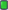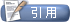MATLAB中国论坛|MATLAB爱好者之家—不仅仅是MATLAB![MATLAB信号处理] 滤波器设计问题，跪求大佬相助，多谢
 用户名 记住信息 密码
 注册账号 论坛帮助 会员列表 日历事件 搜索 今日新帖 标记版面已读2018-05-29, 11:51 PM #1 初级会员   注册日期: 2018-05-29 年龄: 21 帖子: 1 感谢他人: 0 有 0 帖获得 0 感谢 声望力: 0滤波器设计问题，跪求大佬相助，多谢 要求用双线性变换法设计一个butterworth数字高通滤波器，Wp=0.11*pi，Ws=0.0925*pi，Ap=1，As=50，我用这个代码： Wp=0.11*pi; Ws=0.0925*pi; Ap=1; As=20; T=2;Fs=1/T; wp=2*tan(Wp/2)/T;ws=2*tan(Ws/2)/T;wp1=1/wp;ws1=1/ws; [N,wc]=buttord(wp1,ws1,Ap,As,'s'); [num,den]=butter(N,wc,'s'); [numa,dena]=lp2hp(num,den,1); [numd,dend]=bilinear(numa,dena,Fs); w=linspace(0,pi,1024); h=freqz(numd,dend,w); plot(w/pi,20*log10(abs(h)));然后会出现这个问题：矩阵接近奇异值，或者缩放错误。 请问这是什么原因，该如何解决，或者可以用别的什么代码实现吗？ 多谢！主题工具显示可打印版本邮寄本页给好友 显示模式平板模式切换到混合模式切换到树形模式发帖规则 您不可以发表新主题 您可以发表回复 您不可以上传附件 您不可以编辑自己的帖子 启用 BB 代码 论坛启用 表情符号 论坛启用 [IMG] 代码 论坛禁用 HTML 代码Trackbacks are 禁用 Pingbacks are 禁用 Refbacks are 启用 版面规则

 -- 简体中文 -- 繁體中文 -- English (US) 联系我们 - MATLAB中国论坛|MATLAB爱好者之家 - 论坛存档 - 返回顶端